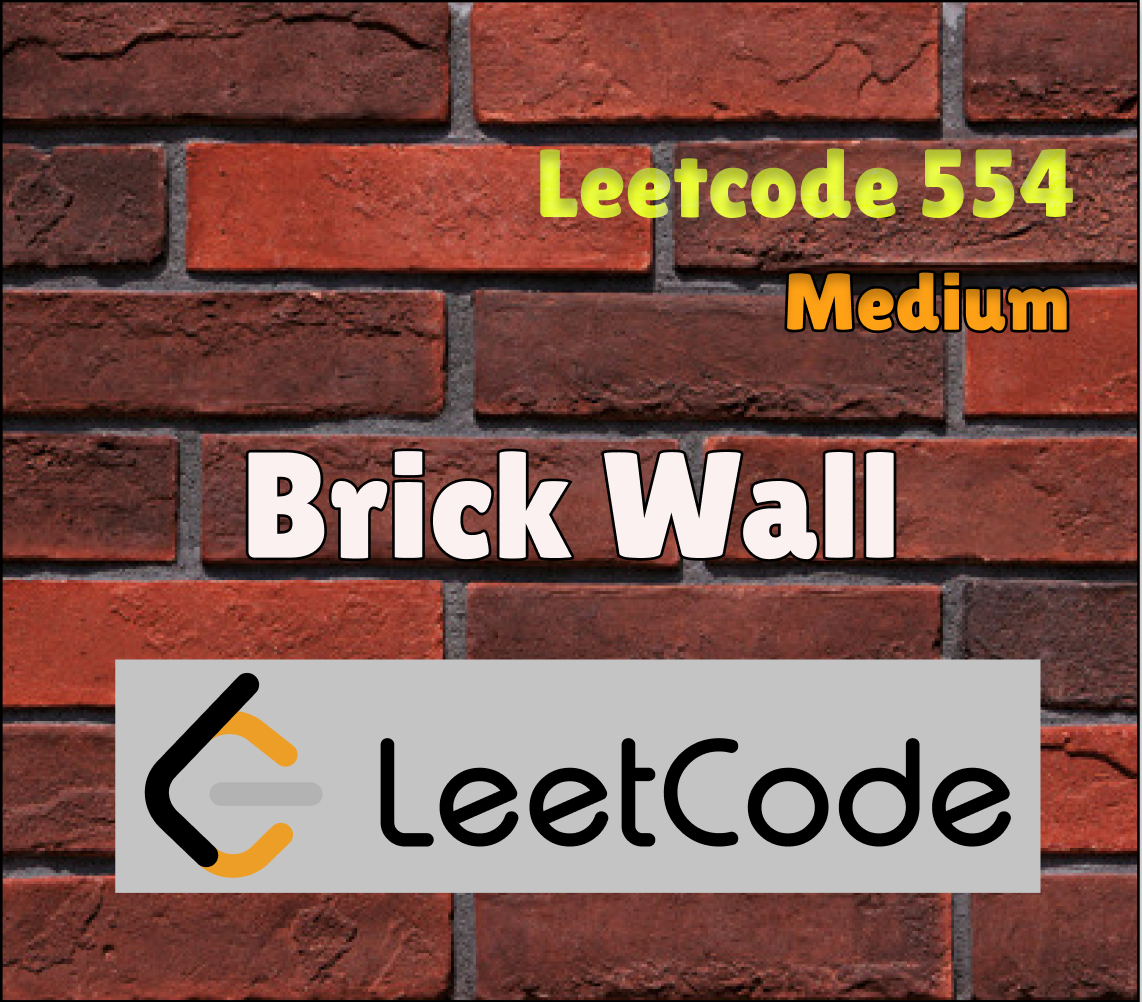# Swift Leetcode Series: Brick Wall

## Check out the Swift solution for Leetcode 554April Leetcode Challenge : Day 22

You can also read the full story on The Swift Nerd blog with the link above.

# Problem Statement

There is a brick wall in front of you. The wall is rectangular and has several rows of bricks. The bricks have the same height but different width. You want to draw a vertical line from the top to the bottom and cross the least bricks.

The brick wall is represented by a list of rows. Each row is a list of integers representing the width of each brick in this row from left to right.

If your line go through the edge of a brick, then the brick is not considered as crossed. You need to find out how to draw the line to cross the least bricks and return the number of crossed bricks.

You cannot draw a line just along one of the two vertical edges of the wall, in which case the line will obviously cross no bricks.

Example:

`Input: [[1,2,2,1],        [3,1,2],        [1,3,2],        [2,4],        [3,1,2],        [1,3,1,1]]Output: 2Explanation:`

# Constraints

1. The width sum of bricks in different rows are the same and won’t exceed INT_MAX.
2. The number of bricks in each row is in range [1,10,000]. The height of wall is in range [1,10,000]. Total number of bricks of the wall won’t exceed 20,000.

# Solution

The problem is easy if we can understand what you actually need to calculate. This is an example of greedy problem and we need to minimise the number of crossed bricks (or pass bisect through them). Since the bricks are of different length and they would be stack horizontally with respect to other layers of bricks, points at which bricks end coincide would way. Focus on the small portion of the picture to understand better.

We bricks which end at the same vertical line would need to have the same offset from the left and the right. Offset can be calculated by adding the lengths of all bricks from start to the current brick. We can maintain a dictionary to store the frequencies of the offsets. Since one offset can occur only once in a single brick row, the count value would represent the number of bricks ending at that distance.

# Prefix Sum

To calculate the offsets, we need to calculate prefix sums for every row. This can be simply done by initialising a row sum variable and iterating through brick array and adding the current brick in the sum variable. After calculating the prefix sum we need to increase the frequency in the dictionary. Beware that we can not take the start and the end points in consideration (since all bricks start and end on the common vertical line, so answer would be always zero on those two lines). While calculating the prefix sum, we need to ignore the last brick in every row, so that its prefix sum won’t be calculated.

# Greedy Approach

The problem is a classical greedy problem as need to minimise/maximise some quantities to get the result. In order for crossing minimum bricks, we need to maximise the bricks ending at a common vertical line. The answer would be finally the difference between the total rows and max rows with a common line.

`min_crossed_bricks = total_brick_rows - max_rows_with_common_endpoint`

# Complexity Analysis

Since we are iterating through every brick, then operations would be equal to the total number of bricks.

Time = O(N * M) where N rows and M is the Max number of bricks possible in a single row.

Space = O(N * M)

Thank you for reading. If you liked this article and found it useful, share and spread it like wildfire!

You can find me on TheSwiftNerd | LinkedIn | Github

--

--